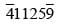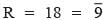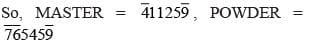Olympiad Test: Coding – Decoding - 2

# Olympiad Test: Coding – Decoding - 2 - Class 6

Test Description

## 20 Questions MCQ Test Science Olympiad Class 6 - Olympiad Test: Coding – Decoding - 2

Olympiad Test: Coding – Decoding - 2 for Class 6 2023 is part of Science Olympiad Class 6 preparation. The Olympiad Test: Coding – Decoding - 2 questions and answers have been prepared according to the Class 6 exam syllabus.The Olympiad Test: Coding – Decoding - 2 MCQs are made for Class 6 2023 Exam. Find important definitions, questions, notes, meanings, examples, exercises, MCQs and online tests for Olympiad Test: Coding – Decoding - 2 below.
 1 Crore+ students have signed up on EduRev. Have you?
Olympiad Test: Coding – Decoding - 2 - Question 1

### If CONCEPT is written as unmulqr and FRIEND is written as ysglmt, then how is PREDICT written in that code?

Detailed Solution for Olympiad Test: Coding – Decoding - 2 - Question 1

Letter : C O N E P T F R I D
Code : u n m l q r y s g t
The code for PREDICT is qsltgur.

Olympiad Test: Coding – Decoding - 2 - Question 2

### In a certain code, FIRE is written as QHOE and MOVE as ZMWE. Following the same rule of coding what should be the code for the word OVER?

Detailed Solution for Olympiad Test: Coding – Decoding - 2 - Question 2

Letter: F I R E M O V
Code: Q H O E Z M W
The code for OVER is MWEO.

Olympiad Test: Coding – Decoding - 2 - Question 3

### If WORK is coded as 4 – 12 – 9 – 16, then how will you code WOMAN?

Detailed Solution for Olympiad Test: Coding – Decoding - 2 - Question 3

Each letter is coded by the numeral obtained by subtracting from 27 then numeral denoting the position of the letter in the English alphabet. W, O, M, A, N are 23rd, 15th, 13th, 1st and 14th letters. So their codes are (27 – 23), (27 – 15), (27 – 13), (27 – 1), (27 – 14)
i.e. 4, 12, 14, 26, 13 respectively.

Olympiad Test: Coding – Decoding - 2 - Question 4

If GO = 32, SHE = 49, then SOME will be equal to

Detailed Solution for Olympiad Test: Coding – Decoding - 2 - Question 4

In the given code, Z = 1, Y = 2, X = 3, ……. C = 24, B = 25, Z = 26.
So, GO = 20 + 12 = 32 and SHE = 8 + 19 + 22 = 49.
Similarly, SOME = S + O + M + E = 8 + 12 + 14 + 22 = 56.

Olympiad Test: Coding – Decoding - 2 - Question 5

If ZIP = 198 and ZAP = 246, then how will you code VIP?

Detailed Solution for Olympiad Test: Coding – Decoding - 2 - Question 5

Taking Z = 2, Y = 3, …., N = 14, ……, B = 26,
A = 27
ZIP
= (Z + I + P) × 6 = (2 + 19 + 12) × 6 = 33 × 6
= 198
VIP
= (V + I + P) × 6 = (6 + 19 + 12) × 6 = 37 × 6
= 222

Olympiad Test: Coding – Decoding - 2 - Question 6

If MASTER is coded as, then POWDER will be coded as.

Detailed Solution for Olympiad Test: Coding – Decoding - 2 - Question 6

Let A = 1, B = 2, C = 3, ……, Z = 26. Now, M = 13 = 4 (Remainder obtained after dividing by 9).
S = 19 = 1 (Remainder obtained after dividing by 9 twice)
T = 20 = 2 (Remainder obtained after dividing by 9 twice)(Remainder obtained after dividing by 9)Olympiad Test: Coding – Decoding - 2 - Question 7

In a certain code, BRAIN is written as * % ÷ # × and TIER is written as \$ # + %. How is RENT written in that code?

Detailed Solution for Olympiad Test: Coding – Decoding - 2 - Question 7

Letter : B R A I N T E
Code : ∗  % ÷ # × \$ +
The code for RENT is % + × \$

Olympiad Test: Coding – Decoding - 2 - Question 8

If DELHI is coded as 73541 and CALCUTTA as 82589662, how can CALICUT be coded?

Detailed Solution for Olympiad Test: Coding – Decoding - 2 - Question 8

Letter: D E L H I C A U T
Code: 7 3 5 4 1 8 2 9 6
The code for CALICUT is 8251896.

Olympiad Test: Coding – Decoding - 2 - Question 9

If NOIDA is written as 39658, how will INDIA be written?

Detailed Solution for Olympiad Test: Coding – Decoding - 2 - Question 9

Letter : N O I D A
Code : 3 9 6 5 8
The code for INDIA is 63568.

Olympiad Test: Coding – Decoding - 2 - Question 10

If ENGLAND is written as 1234526 and FRANCE is written as 785291, how is GREECE coded?

Detailed Solution for Olympiad Test: Coding – Decoding - 2 - Question 10

Letter: E N G L A D F R C
Code: 1 2 3 4 5 6 7 8 9
The code for GREECE is 381191.

Olympiad Test: Coding – Decoding - 2 - Question 11

If REQUEST is written as S2R52TU, then how will ACID be written?

Detailed Solution for Olympiad Test: Coding – Decoding - 2 - Question 11

Vowels A, E, I, O, U are coded as 1, 2, 3, 4, 5 respectively. Each of the consonants in the word is moved one step forward to give the corresponding letter of the code so, the code for ACID becomes ID3E.

Olympiad Test: Coding – Decoding - 2 - Question 12

In a certain code, DESK is written as #. 52, RIDE is written as % 7# . how is RISK written in that code?

Detailed Solution for Olympiad Test: Coding – Decoding - 2 - Question 12

Letter: D E S K R I
Code: # 5 2 % 7
The code for RISK is % 752.

Olympiad Test: Coding – Decoding - 2 - Question 13

In a certain code, EAT is written as 318 and CHAIR is written as 24156. What will TEACHER be written as?

Detailed Solution for Olympiad Test: Coding – Decoding - 2 - Question 13

Letter: E A T C H I R
Code: 3 1 8 2 4 5 6
The code for TEACHER is 8312436.

Olympiad Test: Coding – Decoding - 2 - Question 14

In a certain code, DEAF is written as 3587 and FILE is written as 7465. How is IDEAL written in that code?

Detailed Solution for Olympiad Test: Coding – Decoding - 2 - Question 14

Letter: D E A F I L
Code: 3 5 8 7 4 6
The code for IDEAL is 43586.

Olympiad Test: Coding – Decoding - 2 - Question 15

If DRIVER = 12, PEDESTRIAN = 20, ACCIDENT = 16, then CAR = ?

Detailed Solution for Olympiad Test: Coding – Decoding - 2 - Question 15

CAR = (Number of letters in CAR) × 2 = 3 × 2 = 6

Olympiad Test: Coding – Decoding - 2 - Question 16

If ‘Oranges’ are ‘apples’, ‘bananas’ are ‘apricots’, ‘apples’ are ‘chillies’, ‘apricots’ are ‘oranges’ and chillies are ‘bananas’, then which of the following are green in colour?

Detailed Solution for Olympiad Test: Coding – Decoding - 2 - Question 16

‘Chillies’ are green in colour and as given, ‘chillies’ are ‘bananas’. So, ‘bananas’ are green in colour.

Olympiad Test: Coding – Decoding - 2 - Question 17

If ‘sky’ is ‘star ’, ‘star ’ is ‘cloud’, ‘cloud’ is earth, ‘earth’ is ‘tree’ and ‘tree’ is ‘book’, then where do the birds fly?

Detailed Solution for Olympiad Test: Coding – Decoding - 2 - Question 17

Birds fly in the ‘sky’ and as given, ‘sky’ is ‘star’. So, birds fly in the ‘star’.

Olympiad Test: Coding – Decoding - 2 - Question 18

If ‘blue’ means ‘green’, ‘green’ means ‘white’, ‘white’ means ‘yellow’ means ‘black’, ‘black’ means ‘red’ and ‘red’ means ‘brown’, then what is the colour of milk?

Detailed Solution for Olympiad Test: Coding – Decoding - 2 - Question 18

The colour of milk is ‘white’. But, as given ‘green’ means ‘white’. So, the colour of milk is green.

Olympiad Test: Coding – Decoding - 2 - Question 19

If ‘eraser ’ is called ‘box’, ‘box’ is called ‘pencil’, ‘pencil’ is called ‘sharpener’ and ‘sharpener’ is called ‘bag’, what will a child write with?

Detailed Solution for Olympiad Test: Coding – Decoding - 2 - Question 19

A child will write with a ‘pencil’ and ‘pencil’ is called sharpener. So, a child will write with a ‘sharpener’.

Olympiad Test: Coding – Decoding - 2 - Question 20

If ‘dust’ is called ‘air ’, ‘air ’ is called ‘fire’, ‘fire’ is called ‘water’, ‘water’ is called ‘colour’, ‘colour’ is called ‘rain’ and ‘rain’ is called ‘dust’, then where do fish live?

Detailed Solution for Olympiad Test: Coding – Decoding - 2 - Question 20

Fishes l ive in ‘water’ and as given, ‘water’ is called ‘colour’. So, fishes live in ‘colour’.